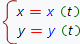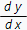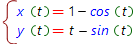# Parametric derivative online calculator

Let's define function by the pair of parametric equations:, and atb,

where x(t), y(t) are differentiable functions and x'(t)0. Then the derivativeis defined by the formula:, and atb,

where- the derivative of the parametric equation y(t) by the parameter t and- the derivative of the parametric equation
x(t), by the parameter t.

Our online calculator finds the derivative of the parametrically derined function with step by step solution. The example of the step by step solution can be found here.

## Choose expression input type:

Expression input type:x(t)=
y(t)=